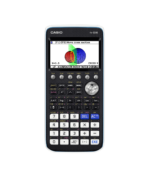Solve Equations Archives - Casio Calculators

# Solve Equations

Displaying Resources

FilterHow to Video
fx-CG50 A-Level IB
Quick Skill: SolveN – Using List Values

How to extract List values to perform operations in the Run-Matrix app. You will...How to Video
fx-CG50 A-Level GCSE IB
Quick Skill: Simultaneous Equations – Using Equation Solutions

How to extract the solutions to a system of linear simultaneous equations found ...How to Video
fx-CG50 A-Level GCSE IB
Quick Skill: Polynomials – Using Equation Results

How to extract a polynomial's solutions found using the Equation app. You will n...How to Video
fx-CG50 A-Level IB
Trigonometric Equations – Solving Numerically

How to use the SolveN function to solve trigonometric equations with exact value...How to Video
fx-CG50 GCSE IB A-Level
Simultaneous Equations – Solving Numerically

How to use Equation to solve simultaneous linear equations in two variables nume...How to Video
fx-CG50 A-Level
Simultaneous Equations – Solving Harder Equations Graphically

How to solve a simultaneous linear and quadratic equation in both x and y graphi...How to Video
fx-CG50 GCSE IB A-Level
Simultaneous Equations – Solving Graphically

How to solve two simultaneous linear, or linear and quadratic, equations using g...How to Video
fx-CG50 IB A-Level GCSE

How to use Equation to numerically solve a polynomial equation with real roots....How to Video
A-Level GCSE
Solving Polynomials

How to solve polynomials of order 2, 3 or 4 with exact values. Find vertex of a ...How to Video
A-Level GCSE
Solve Function

How to solve equations numerically by inputting a start value for each solution....How to Video
GCSE A-Level
Simultaneous Equations

How to solve linear simultaneous equations. Rearrange equations to correctly inp...How to Video
A-Level GCSE
Calc Function

How substitute values of x into a function using Calc and store the result....Teach Leaflet
fx-CG50 A-Level
The Newton-Raphson Method

This resource guides learners to see graphically that the intersection with the ...Teach Leaflet
fx-CG50 A-Level
Solve Equations Using Fixed Point Iteration

This resource is an activity for solving equations numerically including the use...Teach Leaflet
fx-CG50 A-Level
Change of Sign Method for Finding Roots

This resource leads learners through the process of finding a root of a cubic eq...Teach Leaflet
fx-CG50 IB A-Level
Trigonometric Equations Using Identities

The activity provides practice in sorting trigonometric equations where more tha...Teach Leaflet
fx-CG50 A-Level GCSE IB
Basic Trigonometric Equations

This is an introductory matching exercise focusing on finding two solutions of t...Teach Leaflet
fx-CG50 A-Level GCSE IB
Simultaneous Linear Equations

This learning resource consists of a learner worksheet to teach how to use the s...Teach Leaflet
fx-CG50 A-Level GCSE IB
Equations and Inequalities

This resource teaches a variety of methods in which the Casio fx-CG50 can be use...How to Leaflet
fx-CG50 A-Level
Trigonometric Equations – Solving Numerically (Worksheet)

Worksheet to accompany the video on how to use the SolveN function to solve trig...